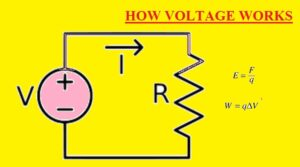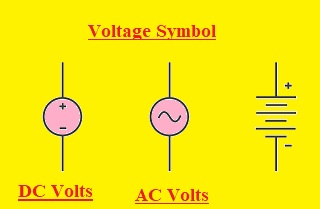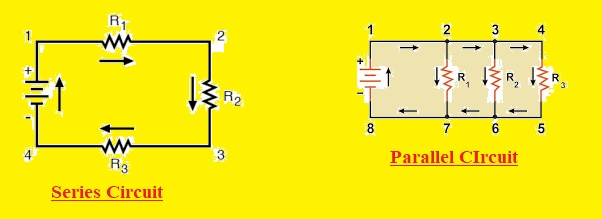Hello, readers welcome to the new post. Today we will discuss HOW VOLTAGE WORKS. Voltage is called electric pressure, electric tension, or potential difference. It is the difference in electric potential between the two points. For static electric field, it is related to the work required per unit charge to move test charge among 2 points. The unit for voltage in the system international unit is volts. In the SI system work per unit, and the charge is called jouls per column.

In this post, we will discuss different parameters to learn the working of volts. So let’s get started with HOW VOLTAGE WORKS.

### What is Voltage

• The force that causes the charges to flow is called voltage. Voltage is also called EMF or electromotive force.
• It is the potential difference between two points when one has a high density of electrons than the other.
• The measuring unit of voltage is volts that are driven from electric potential. Its name is given by the Italian physicist Alessandro Volta who created the chemical battery called voltaic pile in 1799.
• The voltage between points can be generated by the electric charge and through electromotive force or generators, inductors, and transformer
• Potential differences can be created by the electromechanical process like the thermoelectric effect and piezoelectric effect.
• A voltmeter is used for measuring the volts between two points.### What is a VOLTAIC PILE

• There are three different discs are used for the voltaic pile one created with zin, the other with copper, and the third one is created with leather soaked in acid.
• The acid in the leather strip the zinc atoms of the 2 valence electrons. Now there are two electrons deficiency in zinc so it will get two electrons from the copper
• As result, zinc plate has additional electrons and copper plates have fewer electrons. This phenomenon is showing the electric potential energy. The difference between the zinc and copper plate electric potential energy is called voltage
• If two terminals of tower are attached electrons flow from the zinc to copper plate that makes electricity### What is the Definition of Voltage

• Voltage is expressed as potential energy per unit charge

V=E/Q

• V denotes the voltage E is the potential difference in energy Q is the charge of the coulomb
• This expression is used to define the charge and potential energy. One volt is equal to one joule per coulomb of charge

### Voltage Units

• There are two types of units used for voltage Si and Base units

SI units of Voltage

• The volt is the system’s international unit of voltage. It is denoted as V and volt are called the derived unit of voltage.
• The Italian physicist Alessandro Volta who invented the voltaic pile has given this unit volt is the honor of his name.

Volts in SI Base Units

• Volt is the electric potential difference between 2 points in a circuit where one joule of energy per coulomb dissipated of charge passes through the electric circuit.
• 1Volt= potential energy/Charge= 1J/1C= kgm2/As3

### Voltage Formula

• The formula of voltage is shown hereor

• According to Ohm’s Law, the voltage formula is
• V= Ix R

### Voltage Symbol

• The symbol for both AC and DC volts are shown here### Difference Between Volt and Current

• The main differences between volts and current are discussed below.
• Voltage is the potential difference between two points in an electric field and current is the flow of charges between two points.
• SI unit of voltage is volt and amper is unit of current
• Voltage is denoted with V and current with I
• a voltmeter is used for voltage measuring and an ammeter is used for the current measure
• The voltage formula is W/Q and the current formula is Q/t
• 1 Volt= 1J/1C and 1 amper= 1 C/1S
• For parallel circuits, voltage is the same and for series circuit current is same
• Voltage has a magnetic field and current has an electrostatic field

### What is a Common Voltage?

• The common voltage is known as the voltage level or rating of electrical instruments.
• Here some values of common voltage for different devices is given
• For USB five volts of dc is a common voltage
• The lead acid battery has 12 volts dc common volts
• HVAC has 110kv to 1200 kV AC
• High-speed trains have 12kv and 50-kV ac
• Single-cell have 1.2 volts

### SERIES AND PARALLEL CIRCUITS

• Normally there are two types of circuits combination used in electrical systems parallel and series combination
• The series circuit has connected all components in series and the same value of current flow through them. While different values of volts will be across every component of the circuit. In this combination, there is voltage dissipation according to the resistance of every load. The resistance of each component is summed up and the net voltage loss will be equal to the EMF of the power supply
• While in parallel combination volts are the same for each component and the current will have a different value#### Voltage Applications

• Common applications of voltage is listed here
• Electrostatic printing
• Testing of electric devices
• Food and beverage testing
• Mass spectroscopy
• Electrostatic coating of material
• Cosmology study of space
• Jet propulsion laboratory

That is all about HOW VOLTAGE WORKS all details has explained. If you have any query ask here